Courses

# Psychrometric Chart And Its Application To Air-Conditioning - 3

## 20 Questions MCQ Test Thermodynamics | Psychrometric Chart And Its Application To Air-Conditioning - 3

Description
This mock test of Psychrometric Chart And Its Application To Air-Conditioning - 3 for Mechanical Engineering helps you for every Mechanical Engineering entrance exam. This contains 20 Multiple Choice Questions for Mechanical Engineering Psychrometric Chart And Its Application To Air-Conditioning - 3 (mcq) to study with solutions a complete question bank. The solved questions answers in this Psychrometric Chart And Its Application To Air-Conditioning - 3 quiz give you a good mix of easy questions and tough questions. Mechanical Engineering students definitely take this Psychrometric Chart And Its Application To Air-Conditioning - 3 exercise for a better result in the exam. You can find other Psychrometric Chart And Its Application To Air-Conditioning - 3 extra questions, long questions & short questions for Mechanical Engineering on EduRev as well by searching above.
QUESTION: 1

### In an air-conditioning plant the refrigeration load on the coil is 100 TR. The mass and enthalpy of air leaving the coil are 420 kg/minute and 40 kJ/kg respectively. What will be the enthalpy of the air at the inlet to the coil under these conditions.

Solution:

100 TR = 100 X 210 = 21000 kJ/min
Now refrigeration capacity = m (hi - hf) 21000 = 420 (hi - 40)
hi = 90 kJ/kg

QUESTION: 2

Solution:
QUESTION: 3

### Match List-I with List-ll and select the correct answer using the codes given below the lists: List-I A. Milk Chilling plant B. Textile Mills C. Biological process industry List-ll 1. Strict control of temperature and humidity 2. Strict control of humidity 3. Strict control of temperature Codes:     A B C (a) 3 2 1 (b) 1 2 3 (c) 2 3 1 (d) 2 1 3

Solution:
• Strict control of temperature metrological laboratories, precision m/c shops, computer centres etc.
• Relative humidity control-paper and textile mills.
• Strict control of both-chemical and biological industries.
QUESTION: 4

In a counter flow cooling tower, warm water enters at temperature of Tw. The dry bulb and wet bulb temperatures of air entering the tower are TDBT and TWBT respectively. The maximum temperature of water at the exit of the cooling tower is

Solution:
QUESTION: 5

The latent heat load in an auditorium is 25% of the sensible heat load. The value of sensible heat factor (SHF) is equal to

Solution:

Given, LH = 0.25 (SH)
Sensible heat factor,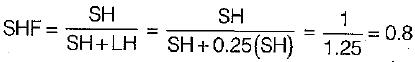QUESTION: 6

Room sensible heat factor will be equal to, if room sensible heat and room latent heat are equal to 70 kW and 30 kW respectively

Solution:

Given room sensible heat (RSH) = 70 kW
Room latent heat (RLH) = 30 kW
Room sensible heat factor (RSHF)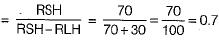Room latent factor heat (RLHF)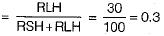QUESTION: 7

Which of the following properties increase(s) during sensible heating of air-water vapour mixture?
1. Relative humidity
2. Humidity ratio
3. Wet bulb temperature
4. Specific enthalpy of air-vapour mixture
Q. Select the correct answer from the codes given below:

Solution:

Effect of sensible heating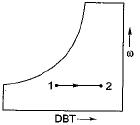1. Dry bulb temperature increases
2. Wet bulb temperature increases
3. Relative humidity decreases
4. Specific enthalpy increases
5. Specific humidity remains constant

QUESTION: 8

Atmospheric air at DBT of 15°C enters a heating coil whose surface temperature is maintained at 40°C. The air leaves the heating coil at 25°C. What will be the by-pass factor of heating coil?

Solution: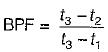Where
t3 = temperature of heating coil = 40°C
t2 = exit temperature of air = 25°C
t1 = temperature of air at inlet = 15°C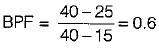QUESTION: 9

In the case of a cooling coil with non-zero by pass factor, the apparatus dew point temperature lies at the intersection point of

Solution: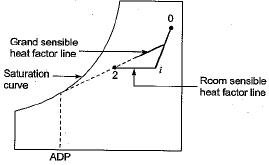Hence apparatus dew point temperature lies at the intersection point of grand sensible heat factor line and saturation curve.

QUESTION: 10

If moist air is sensibly cooled above its dew point which of the following statements are correct:
1. Relative humidity decreases
2. Wet bulb temperature decreases
3. Wet bulb temperature increases
4. Humidity ratio remains constant
Q. Select the correct answer using the codes given below:

Solution: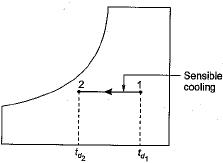When moist air is sensibly cooled
1. Its relative humidity increases
2. Its wet bulb temperature decreases
3. Its enthalpy decreases
4. Its humidity ratio remains constant

QUESTION: 11

Air (at atmospheric pressure) at a dry bulb temperature of 40°C and wet bulb temperature of 20°G is humidified in an air washer operating with continuous water recirculation. The wet bulb depression (i.e. The difference between the dry bulb and wet bulb temperatures) at the exit is 25% of that at the inlet. The dry bulb temperature at the exit of the air washer is . closest to

Solution:

Air (At atmospheric pressure) DBT = 40°C (wet bulb depression)exit = 25% (wet bulb dep)in|et
tdbt - 20 = 0.25(20)
(tabt)exit  = 25°C

QUESTION: 12

Which one of the following is correct for the process 1-2 shown below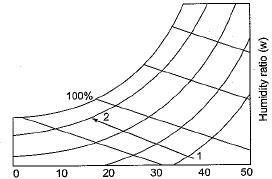Solution:

Since constant enthalpy lines are parallel or coinciding with constant WBT lines. The line shown on the figure is along constant enthalpy.

QUESTION: 13

The supply air temperature is 15°C and apparatus dew point is 12°C for the coding coil with by pass factor of 0.15. What is the temperature at inlet of cooling coil

Solution: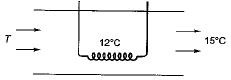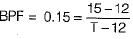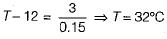QUESTION: 14

Various psychrometric processes are shown in the figure below: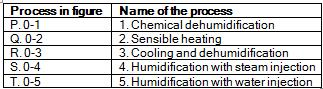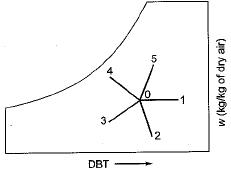The matching pair are

Solution:
QUESTION: 15

The following statements are concerned with psychrometric chart:
1. Constant relative humidity lines are uphill straight lines to the right
2. Constant wet bulb temperature lines are downhill straight lines to the right
3. Constant specific volume lines are downhill straight lines to the right
4. Constant enthalpy fines are coincident with constant wet bulb temperature lines
Q. Which of the statements are correct?

Solution:
QUESTION: 16

Water at 42°C is sprayed into a stream of air at atmospheric pressure, dry bulb temperature of 40°C and a wet bulb temperature of 20°C. The air leaving the spray humidifier is not saturated.
Q. Which of the following statements is true?

Solution:

Given, water spraying temperature = 42°C
Dry bulb temperature Td = 40°C
Wet bulb temperature Tw = 20°C
Here we see that
Water spraying temperature > Td

QUESTION: 17

For air at a given temperature, as the relative humidity is increased isothermally,

Solution:
QUESTION: 18

An air-conditioned room of volume 10 m3 has infiltration of air equivalent of 3 air changes. Density of air is 1.2 kg/m3, specific heat Cp is 1 kJ/kg-K and temperature difference between room and ambient air is 20 K. The sensible heat load due to infiltrated air is

Solution: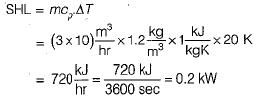QUESTION: 19

A classroom is to be air-conditioned by obtaining the comfort conditions of 22°C DBT ad 55% RH from outdoor conditions of 32°C DBT and 22°C WBT. The weight of outside air supplied is 30 kg/min. The comfort conditions required are achieved first by chemical dehumidification and then by cooling with a cooling coil as shown in the psychrometric chart below. What is the capacity of the dehumidification in kg/hr?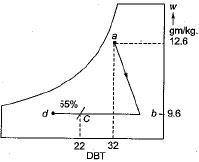Solution:
QUESTION: 20

For an office building the outdoor design conditions are 45°C dbt and humidity ratio of 0.015. The indoor design conditions are 25°C dbt and 0.01 humidity ratio. The supply air state is 15°C dbt and 0.007 humidity ratio. If the supply air flow rate is 1000 m3/min and fresh air flow rate is 100 m3/min, room sensible and room latent heat loads are, respectively

Solution:

SHL = 0.0204 x cmm x ΔT
= 0.0204 x 1000 x (25 - 15) = 204 kW
LHL = 50 cmm x Δw
= 50 x 1000 x (0.01 - 0.007)
= 50 x 1000 x 0.003 = 150 kW